# Non Verbal Reasoning - Image Analysis

### Exercise :: Image Analysis - Section 3

1.

Find out how will the key figure (X) look like after rotation.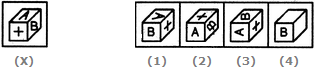A. 1 B. 2 C. 3 D. 4

Explanation:

No answer description available for this question. Let us discuss.

2.

Find out how will the key figure (X) look like after rotation.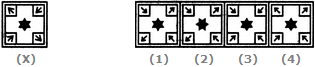A. 1 B. 2 C. 3 D. 4

Explanation:

No answer description available for this question. Let us discuss.

3.

Find out how will the key figure (X) look like after rotation.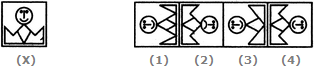A. 1 B. 2 C. 3 D. 4

Explanation:

No answer description available for this question. Let us discuss.

4.

Find out how will the key figure (X) look like after rotation.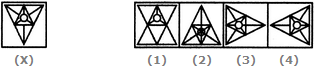A. 1 B. 2 C. 3 D. 4

Explanation:

No answer description available for this question. Let us discuss.

5.

Find out how will the key figure (X) look like after rotation.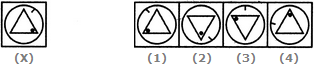A. 1 B. 2 C. 3 D. 4